## Modulus (Elliptic Integral)

A parameter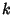used in Elliptic Integrals and Elliptic Functions defined to be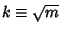, whereis the Parameter. An Elliptic Integral is written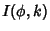when the modulus is used. It can be computed explicitly in terms of Theta Functions of zero argument: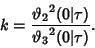(1)

The Real period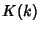and Imaginary period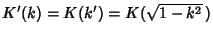are given by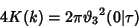(2)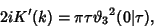(3)

whereis a complete Elliptic Integral of the First Kind and the complementary modulus is defined by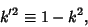(4)

withthe modulus.

See also Amplitude, Characteristic (Elliptic Integral), Elliptic Function, Elliptic Integral, Elliptic Integral Singular Value, Modular Angle, Nome, Parameter, Theta Function

References

Abramowitz, M. and Stegun, C. A. (Eds.). Handbook of Mathematical Functions with Formulas, Graphs, and Mathematical Tables, 9th printing. New York: Dover, p. 590, 1972.